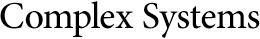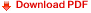## Formal Logic of Cellular AutomataSukanta Das
Department of Information Technology
Indian Institute of Engineering Science and Technology
Shibpur, 711103, India
sukanta@it.iiests.ac.in

Mihir K. Chakraborty
School of Cognitive Science
This paper develops a formal logic, named ${L}_{\mathrm{CA}}$, targeting modeling of one-dimensional binary cellular automata. We first develop the syntax of ${L}_{\mathrm{CA}}$, then give semantics to ${L}_{\mathrm{CA}}$ in the domain of all binary strings. Then the elementary cellular automata and four-neighborhood binary cellular automata are shown as models of the logic. These instances point out that there are other models of ${L}_{\mathrm{CA}}$. Finally it is proved that any one-dimensional binary cellular automaton is a model of the proposed logic.